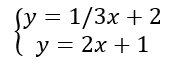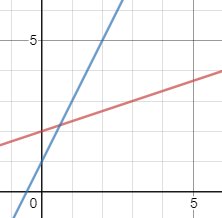# Types of solutions to systems of equations

Topic:
Equations

Below is the graph the following systems of equations to determine the solution. What do you notice about the slopes and y-intercepts of this system?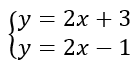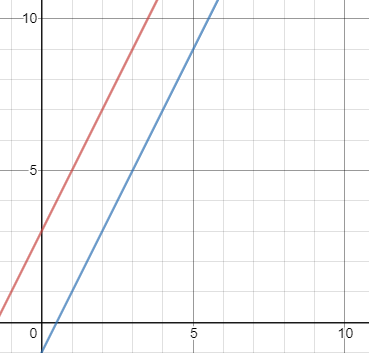Below is the graph the following systems of equations to determine the solution. What do you notice about the slopes and y-intercepts of this system?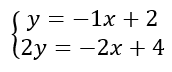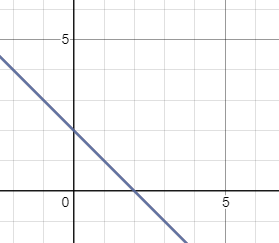Below is the graph the following systems of equations to determine the solution. What do you notice about the slopes and y-intercepts of this system?